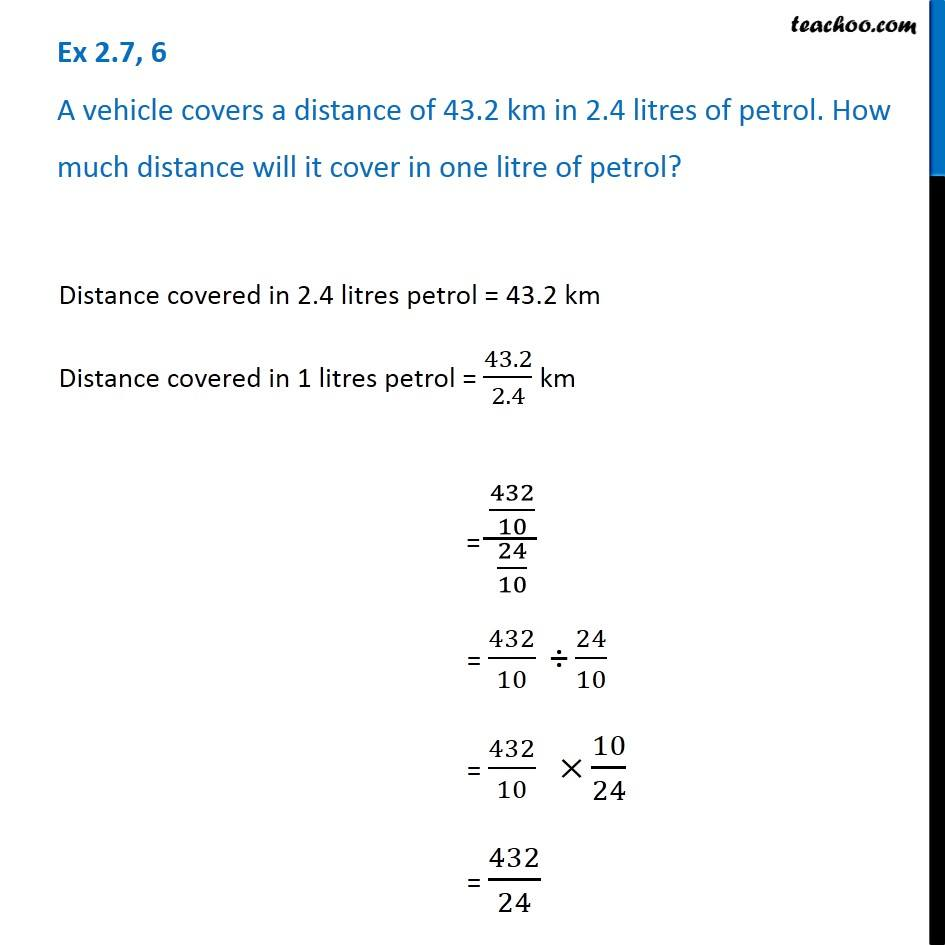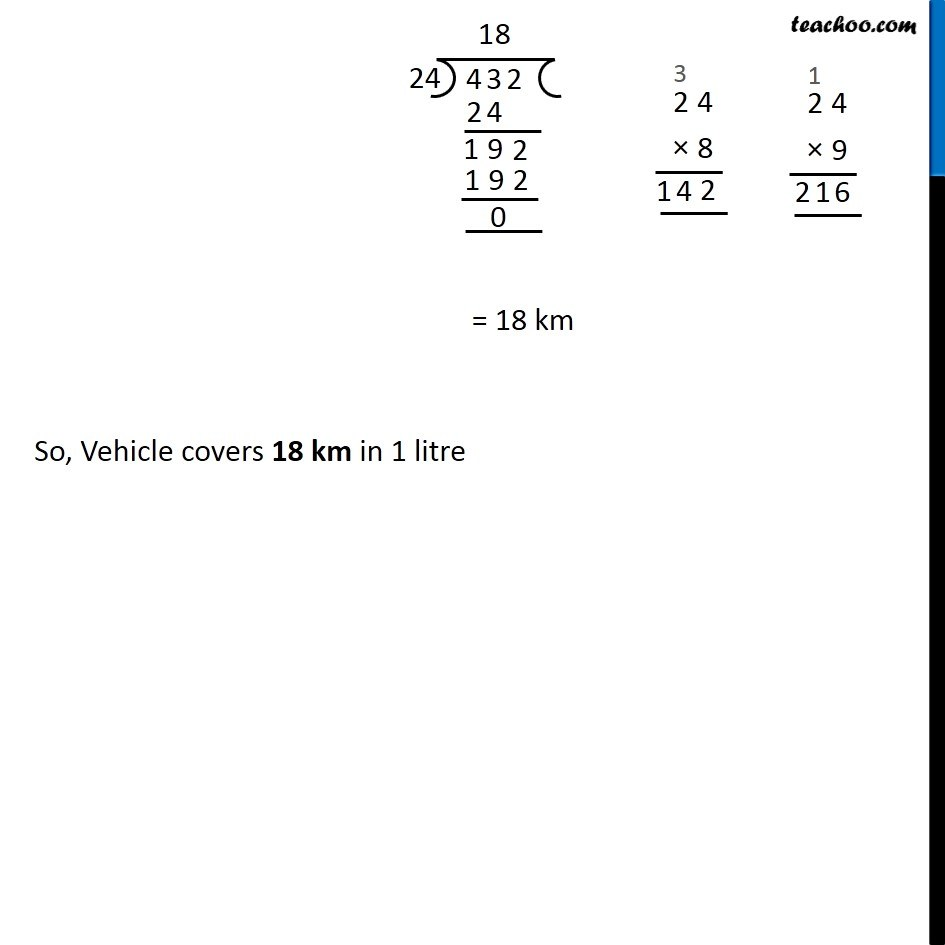1. Chapter 2 Class 7 Fractions and Decimals
2. Concept wise
3. Division of Decimals - Statement Questions

Transcript

Ex 2.7, 6 A vehicle covers a distance of 43.2 km in 2.4 litres of petrol. How much distance will it cover in one litre of petrol? Distance covered in 2.4 litres petrol = 43.2 km Distance covered in 1 litres petrol = 43.2/2.4 km = (432/10)/(24/10) = 432/10 " ÷" 24/10 = 432/10 " "× 10/24 = 432/24 = 18 km So, Vehicle covers 18 km in 1 litre

Division of Decimals - Statement Questions